# Testing Simple Objects CS 5010 Program Design Paradigms

• Slides: 21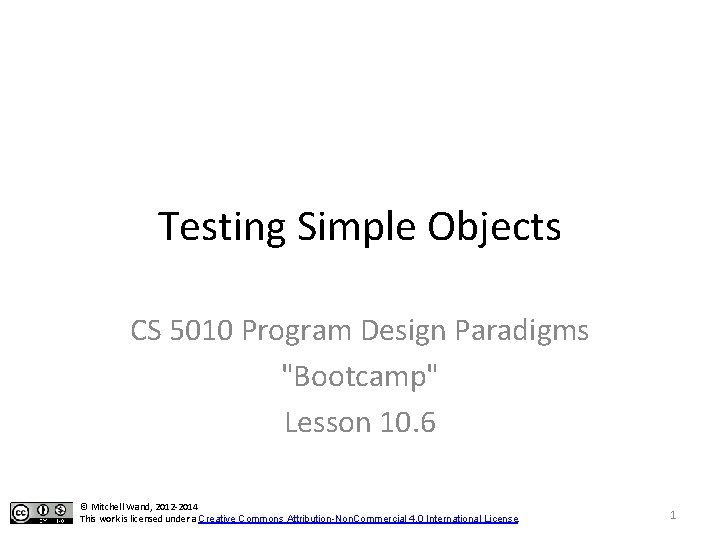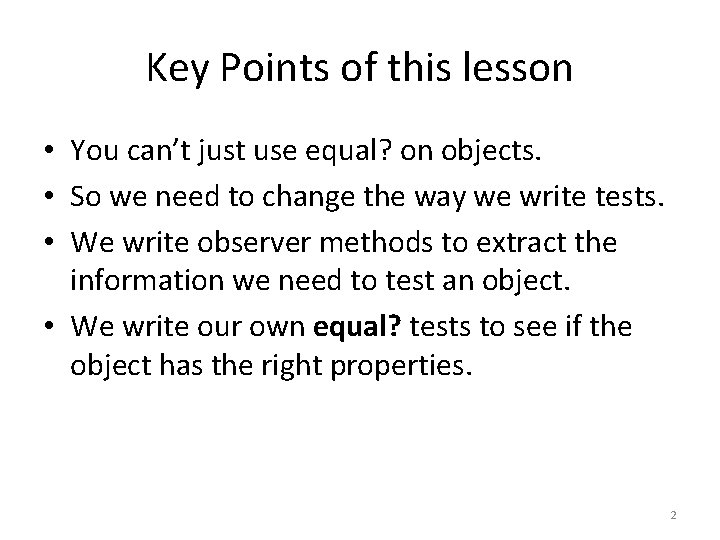Key Points of this lesson • You can’t just use equal? on objects. • So we need to change the way we write tests. • We write observer methods to extract the information we need to test an object. • We write our own equal? tests to see if the object has the right properties. 2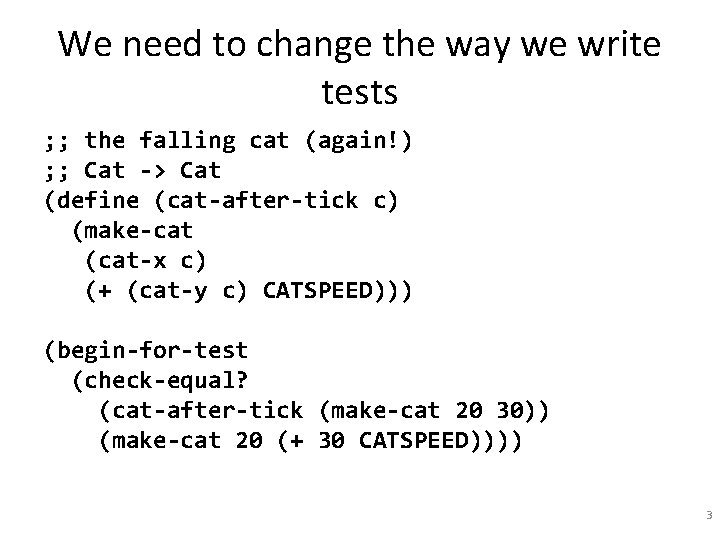We need to change the way we write tests ; ; the falling cat (again!) ; ; Cat -> Cat (define (cat-after-tick c) (make-cat (cat-x c) (+ (cat-y c) CATSPEED))) (begin-for-test (check-equal? (cat-after-tick (make-cat 20 30)) (make-cat 20 (+ 30 CATSPEED)))) 3The OO Cat (define Cat<%> (interface () after-tick )) ; ; a Cat is a (new Cat% [x Int][y Int]) (define Cat% (class* object% (Cat<%>) (init-field x y) ; ; the x and y positions of the center of the ; ; cat (super-new) (define/public (after-tick) (new Cat% [x x][y (+ y CATSPEED)])) ))Testing the OO Cat (begin-for-test (check-equal? (send (new Cat% [x 20][y 30]) after-tick) (new Cat% [x 20][y (+ 30 CATSPEED)]) "Surprise!")) This fails! Why? It has all the right fields.The Big Secret • Here's a secret: objects have identity! • We can have two different objects with the same fields. • In Racket, equal? on objects tests whether its arguments are the same object. 6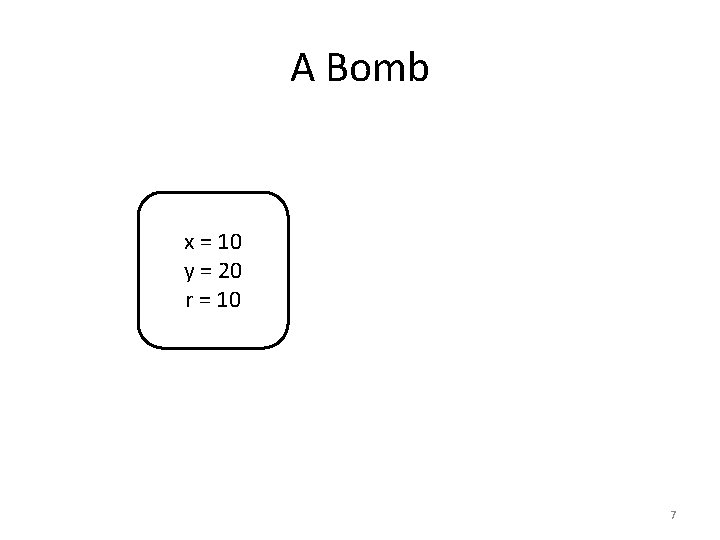A Bomb x = 10 y = 20 r = 10 7One bomb or two? (define b 1 (make-bomb)) (define b 2 b 1) b 1 x = 10 y = 20 r = 10 b 2 (equal? b 1 b 2) = true 8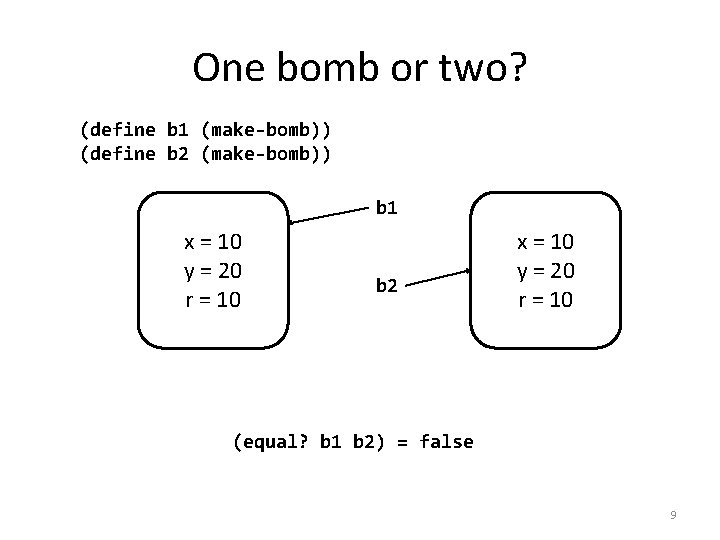One bomb or two? (define b 1 (make-bomb)) (define b 2 (make-bomb)) b 1 x = 10 y = 20 r = 10 b 2 x = 10 y = 20 r = 10 (equal? b 1 b 2) = false 9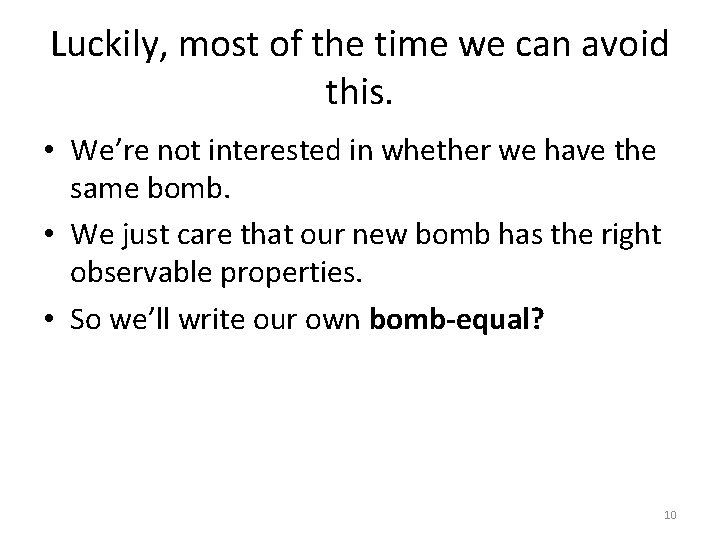Luckily, most of the time we can avoid this. • We’re not interested in whether we have the same bomb. • We just care that our new bomb has the right observable properties. • So we’ll write our own bomb-equal? 10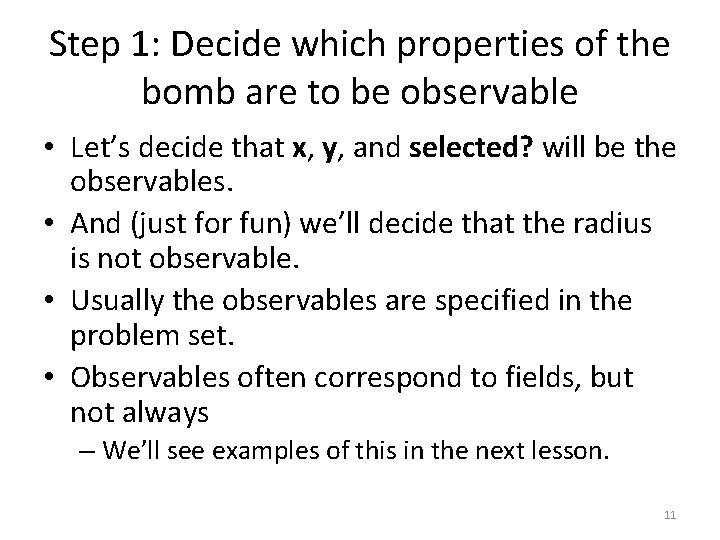Step 1: Decide which properties of the bomb are to be observable • Let’s decide that x, y, and selected? will be the observables. • And (just for fun) we’ll decide that the radius is not observable. • Usually the observables are specified in the problem set. • Observables often correspond to fields, but not always – We’ll see examples of this in the next lesson. 11Step 2: Add observation methods to get the values of these observable quantities ; ; -> Int (define/public (get-x) x) ; ; -> Int (define/public (get-y) y) ; ; -> Boolean (define/public (get-selected? ) 12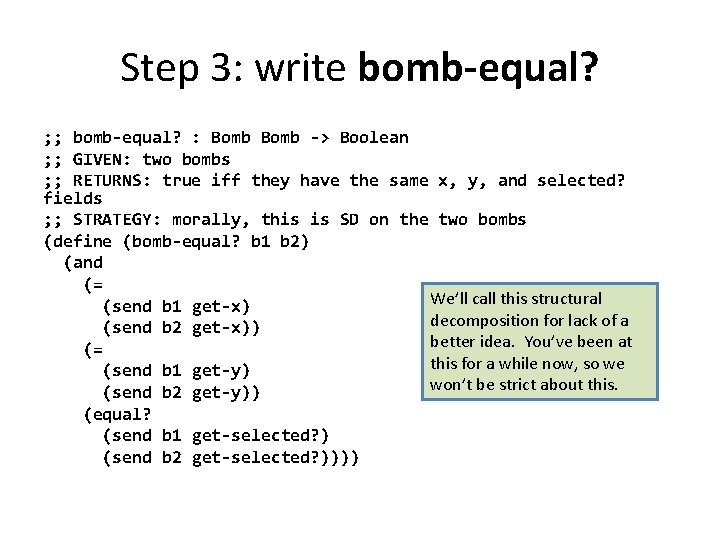Step 3: write bomb-equal? ; ; bomb-equal? : Bomb -> Boolean ; ; GIVEN: two bombs ; ; RETURNS: true iff they have the same x, y, and selected? fields ; ; STRATEGY: morally, this is SD on the two bombs (define (bomb-equal? b 1 b 2) (and (= We’ll call this structural (send b 1 get-x) decomposition for lack of a (send b 2 get-x)) better idea. You’ve been at (= this for a while now, so we (send b 1 get-y) won’t be strict about this. (send b 2 get-y)) (equal? (send b 1 get-selected? ) (send b 2 get-selected? ))))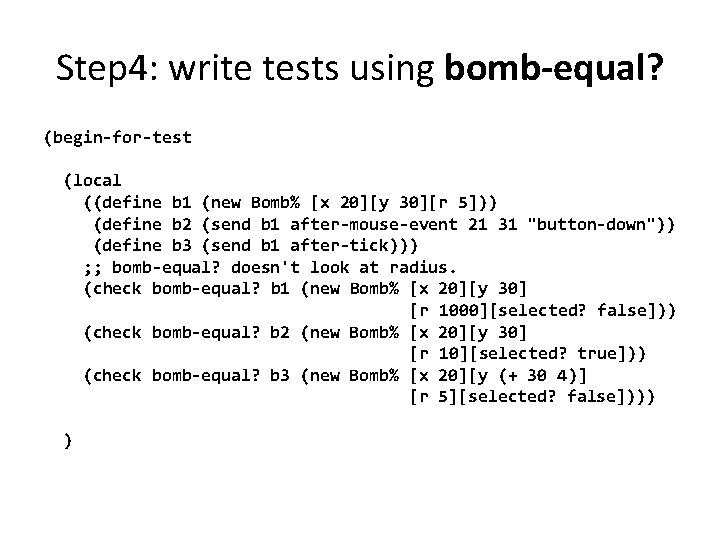Step 4: write tests using bomb-equal? (begin-for-test (local ((define b 1 (new Bomb% [x 20][y 30][r 5])) (define b 2 (send b 1 after-mouse-event 21 31 "button-down")) (define b 3 (send b 1 after-tick))) ; ; bomb-equal? doesn't look at radius. (check bomb-equal? b 1 (new Bomb% [x 20][y 30] [r 1000][selected? false])) (check bomb-equal? b 2 (new Bomb% [x 20][y 30] [r 10][selected? true])) (check bomb-equal? b 3 (new Bomb% [x 20][y (+ 30 4)] [r 5][selected? false]))) )We could write other class-specific equal? tests, too Here we assume that x and y are the observables for Heli. This is reasonable, ; ; Heli -> Boolean (define (heli-equal? heli 1 heli 2) since that is where the heli will be displayed. (and (= (send heli 1 get-x) (send heli 2 get-x)) (= (send heli 1 get-y) (send heli 2 get-y)))) (define (world-equal? w 1 w 2) Here we've used the 2 -argument (and version of andmap, which is (heli-equal? (send w 1 get-heli) available in #lang racket, but not in (send w 2 get-heli)) ISL+Lambda (andmap (lambda (b 1 b 2) (bomb-equal? b 1 b 2)) (send w 1 get-bombs) This test requires that the (send w 2 get-bombs)))) bombs appear in the same order. If we didn’t want order to count, then we’d need something like set-equal?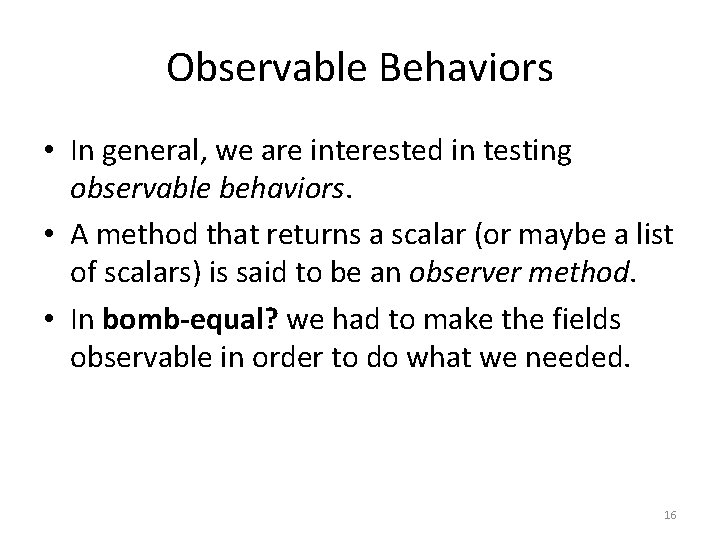Observable Behaviors • In general, we are interested in testing observable behaviors. • A method that returns a scalar (or maybe a list of scalars) is said to be an observer method. • In bomb-equal? we had to make the fields observable in order to do what we needed. 16Observables in the problem sets • In our problem sets, we've required you to provide just enough observables so that our automated testing routines can see if you've solved the problem. • In a test, we create a scenario and then check the observables of the final state. 17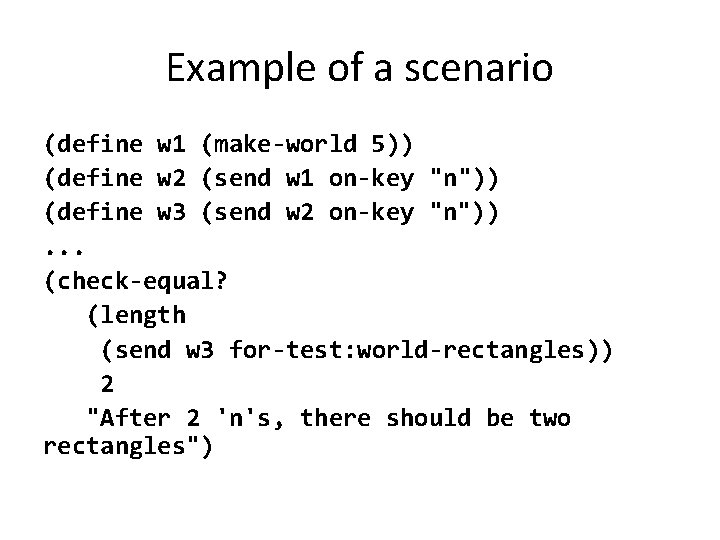Example of a scenario (define w 1 (make-world 5)) (define w 2 (send w 1 on-key "n")) (define w 3 (send w 2 on-key "n")). . . (check-equal? (length (send w 3 for-test: world-rectangles)) 2 "After 2 'n's, there should be two rectangles")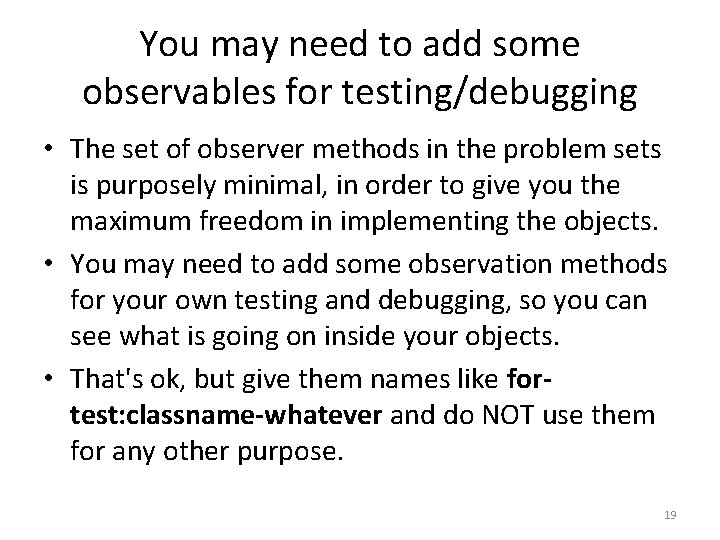You may need to add some observables for testing/debugging • The set of observer methods in the problem sets is purposely minimal, in order to give you the maximum freedom in implementing the objects. • You may need to add some observation methods for your own testing and debugging, so you can see what is going on inside your objects. • That's ok, but give them names like fortest: classname-whatever and do NOT use them for any other purpose. 19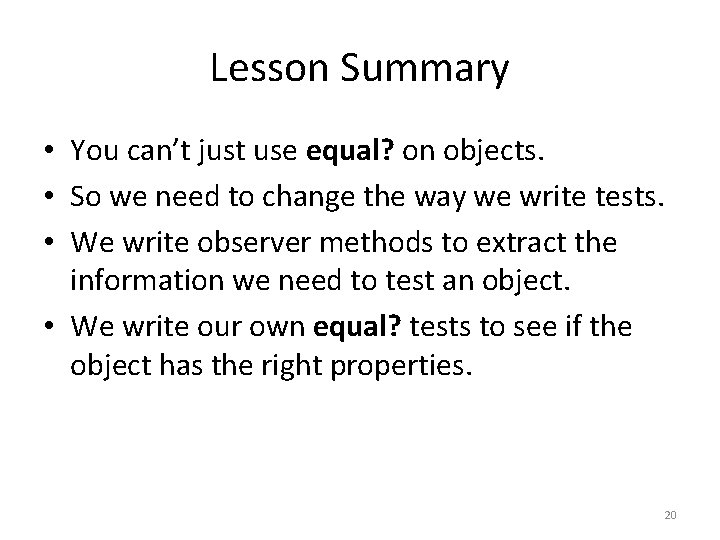Lesson Summary • You can’t just use equal? on objects. • So we need to change the way we write tests. • We write observer methods to extract the information we need to test an object. • We write our own equal? tests to see if the object has the right properties. 20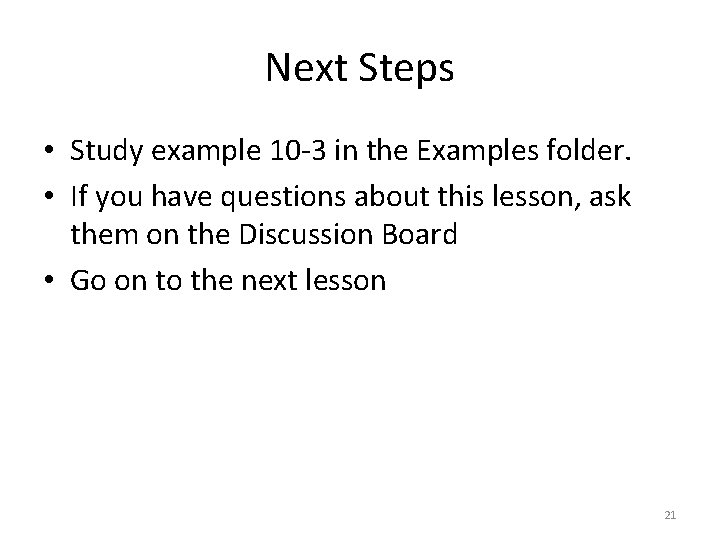Next Steps • Study example 10 -3 in the Examples folder. • If you have questions about this lesson, ask them on the Discussion Board • Go on to the next lesson 21